## Example Questions

2 Next →

### Example Question #541 : Algebra

What are the two solutions to the following quadratic equation?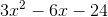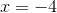andand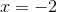andandandandExplanation:

The equation can be factored.

First pull out a common factor of three from each term.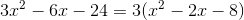.

Now, find the factors of the constant term that when added together result in the middle term.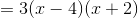The roots are what values will make the equation equal.

Therefore, the answers areand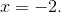2 Next →

### All ACT Math Resources